### Home > CAAC > Chapter 10 > Lesson 10.2.1 > Problem10-53

10-53.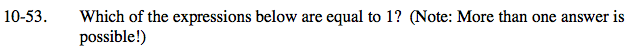Any fraction where the numerator and denominator are the same is equal to 1.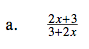Rearrange to see if the numerator and denominator are equal.

$\frac{2x+3}{2x+3}$

The fraction is equal to 1.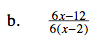Simplify the expression.

$\frac{6x-12}{6x-12}$

The fraction is equal to 1.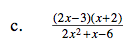Factor the denominator using a generic rectangle and the diamond pattern found in earlier chapters.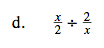Change the expression to a product.

$\left(\frac{x}{2}\right) \left(\frac{x}{2}\right)$

Does this product equal 1?